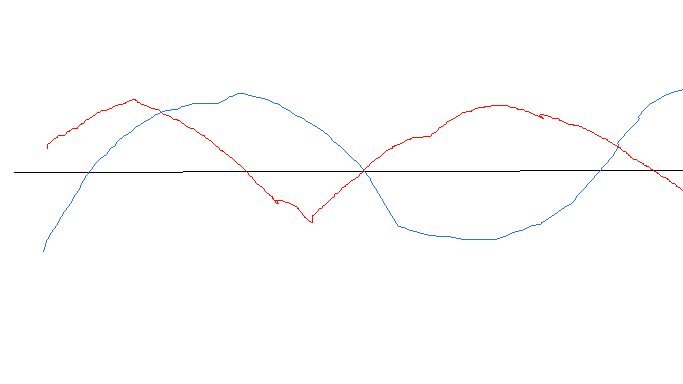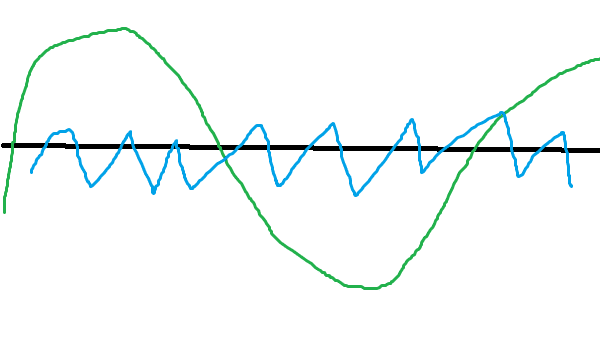Why can't two laser beams from two sources Interfere if they have a difference phase?

I read in the internet that the reason that two laser can't Interference because they most likely have difference phase.
but electromagnetic wave are just change in the electromagnetic filed that goes up and down ,
so mathematically they may Interference little let say that the there is two wave that looks like thiswe can see that the electromagnetic max and min points distance wave would be difference when the both wave are both in the same space so after they would pass each other this change would cancel ?.
and what if they would be point to the same place does the frequency of the wave change ?.
and if the change do cancel when they pass each other how can laser from the same source Interference.
maybe it more fit to the description of light as matter so the photon doesn't change so the electromagnetic filed change only in the specific point so how can two photon form the same source
Interference.
so maybe it because that if the photon come from the same source then they move at the same path always so the change in the electromagnetic filed saves

Last edited:

jbriggs444
Homework Helper
We need a better reference than this in order to intelligently discuss what was said.

•berkeman
Ibix
There are a number of things going on. First, lasers aren't purely monochromatic - they're narrower band than anything else, but they have some frequency spread. Also, they aren't perfectly coherent. They have a coherence length, which is the typical distance across the beam where they remain more or less coherent, and a coherence time, which is the length of time for which you can predict the phase at a point given the phase there now.

Your diagram is for idealised lasers with an infinite coherence time and zero bandwidth. With such things, yes, you could get interference from different lasers. It might be possible to get interference with super-high quality lasers with very long coherence times and narrow bandwidths (never seen it done, but haven't looked either), but not your average laser.

•DaveE, danielhaish and jbriggs444
There are a number of things going on. First, lasers aren't purely monochromatic - they're narrower band than anything else, but they have some frequency spread. Also, they aren't perfectly coherent. They have a coherence length, which is the typical distance across the beam where they remain more or less coherent, and a coherence time, which is the length of time for which you can predict the phase at a point given the phase there now.

Your diagram is for idealised lasers with an infinite coherence time and zero bandwidth. With such things, yes, you could get interference from different lasers. It might be possible to get interference with super-high quality lasers with very long coherence times and narrow bandwidths (never seen it done, but haven't looked either), but not your average laser.
but also if they have difference wave length they would still effect each other . I mean if two levels of two wave that effect the electromagnetic filed meet each other wouldn't them effect each other ?

We need a better reference than this in order to intelligently discuss what was said.
it local source sorry

Ibix
but also if they have difference wave length they would still effect each other . I mean if two levels of two wave that effect the electromagnetic filed meet each other wouldn't them effect each other ?
The electric and magnetic fields add together, sure. But you don't get time-independent patterns unless the frequencies are the same and the phase relationships are stable.

•danielhaish
tech99
Gold Member
I read in the internet that the reason that two laser can't Interference because they most likely have difference phase.
but electromagnetic wave are just change in the electromagnetic filed that goes up and down ,
so mathematically they may Interference little let say that the there is two wave that looks like this
View attachment 269637
we can see that the electromagnetic max and min points distance wave would be difference when the both wave are both in the same space so after they would pass each other this change would cancel ?.
and what if they would be point to the same place does the frequency of the wave change ?.
and if the change do cancel when they pass each other how can laser from the same source Interference.
maybe it more fit to the description of light as matter so the photon doesn't change so the electromagnetic filed change only in the specific point so how can two photon form the same source
Interference.
so maybe it because that if the photon come from the same source then they move at the same path always so the change in the electromagnetic filed saves
Any two waves will interfere, but if they have different frequencies the interference pattern will be moving all the time, usualy too fast to see. It will repeat itself at the difference frequency. If, however, the frequencies are locked, then the inteference pattern will be stationary. If one source then has a fixed phase shift then of course the pattern will be changed or displaced. If two locked sources have random phase variations - we might say the time coherence is reduced - then the interference pattern will be noisy, and in an extreme case might not be discernible.

•DaveE, danielhaish and Ibix
DaveE
Gold Member
Others have describe this, but here's my explanation:

The phase of wave interaction is usually only useful if it is a consistent value. That means that the waves have nearly EXACTLY the same frequency (frequency is the rate of change of phase, BTW). That almost always means the two waves were generated from the same source.

Yes any two waves will interfere with each other. This is just a consequence of the fact that the amplitude of the EM fields is the sum of the waves that are present at any point in space and time. If the frequencies aren't the same then this interference changes so quickly you can only observe the average result. Since visible light has frequencies of 1014 - 1015 Hz. This means that for an interference effect to be stable for 1 second then light frequencies (or wavelengths) must only differ by 1 part in 1017 or so.

•danielhaish
rude man
Homework Helper
Gold Member
Graph ## sin(2\pi f t) + sin[2\pi (f+\Delta f) t] ## on software like excel with ## \Delta f << f ## and you will see your interference pattern.

•danielhaish
so if I see it right the reason
Others have describe this, but here's my explanation:

The phase of wave interaction is usually only useful if it is a consistent value. That means that the waves have nearly EXACTLY the same frequency (frequency is the rate of change of phase, BTW). That almost always means the two waves were generated from the same source.

Yes any two waves will interfere with each other. This is just a consequence of the fact that the amplitude of the EM fields is the sum of the waves that are present at any point in space and time. If the frequencies aren't the same then this interference changes so quickly you can only observe the average result. Since visible light has frequencies of 1014 - 1015 Hz. This means that for an interference effect to be stable for 1 second then light frequencies (or wavelengths) must only differ by 1 part in 1017 or so.
so if understand all answer the reason it cant be done it because they have difference frequency so in every max point in the low frequency wave there is many max point in the high frequency wave so it would looks like thatdoes this diagram describe it correctly and also does to wave with high frequency have higher max point?

DaveE
•